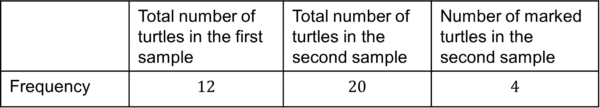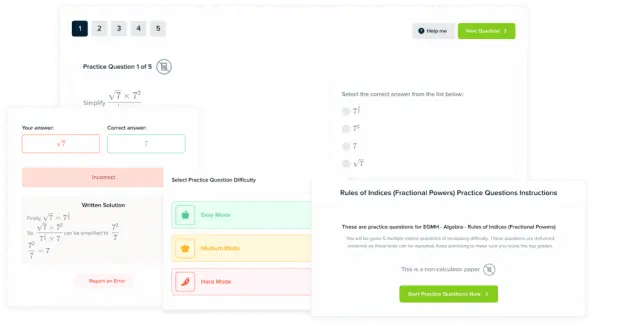# Capture - Recapture

GCSELevel 6-7AQAEdexcelOCR

## Capture – Recapture

Capture-Recapture is a statistical method used to estimate population sizes when it is too difficult to count the entire population.

For example, the number of ants that live in someone’s garden could be estimated. The sampler would capture a specific amount of ants, for example $20$, and mark them with an ethical tag. The ants would then be released, and another time, the sampler would return and capture some more ants. In this second sample, the number of marked ants would be counted and this information would be used to estimate the overall population.

The more marked animals that are recaptured, the closer the overall population size is to the captured amount.

You will need to know how to estimate a population size using this method.

Level 6-7GCSEAQAEdexcelOCR

## Using Capture – Recapture

The key equation for capture-recapture is as follows,

$\dfrac{m}{n}=\dfrac{M}{N}$

Where

$M =$ size of first sample

$N =$ size of total population

$m =$ the number of previously tagged individuals that are recaptured

$n =$ size of second sample

Hence, the equation may be written as,

$\dfrac{\text{Number recaptured}}{\text{Size of second sample}}=\dfrac{\text{Size of first sample}}{\text{Size of population}}$

This can be rearranged to find the size of the total population from the capture-recapture method:

$N = \dfrac{n}{m}\times M\\$

$\text{Size of population}=\dfrac{\text{Size of second sample}}{\text{Number of recaptured}}\times\text{Size of first sample}$

Level 6-7GCSEAQAEdexcelOCR

## Assumptions

As with most statistical methods, there are certain properties we need to assume in order for the capture – recapture method to be as accurate as possible.

These include:

• Marked and unmarked individuals have the same mortality rate (the marking does not cause individuals to be more likely to die)
• The population is closed (there is no death or migration)
• The population must be given time between the first and second sample for the marked and unmarked individuals to fully mix
Level 6-7GCSEAQAEdexcelOCR
Level 6-7GCSEAQAEdexcelOCR

## Example 1

Emerson captures $20$ deer from his local forest and ethically marks them with a tag. He then releases the deer back into the forest. A week later, Emerson returns and captures $50$ deer and counts that $10$ of these deer have the ethical tag he previously put on them.

Use the equation for capture-recapture to work out an estimate for the total deer population in Emerson’s local forest.

[3 marks]

We will use this equation,

$N = \dfrac{n}{m}\times M\\$

So, we need to work out what each of the variables equal,

$N$ (total population) $=$ unknown

$n$ (size of second sample) $=50$ deer

$m$ (number of recaptured) $=10$ deer

$M$ (size of first sample) $=20$ deer

Therefore, the total population size can be estimated as:

$\dfrac{50}{10}\times 20 = 100$ deer

Level 6-7GCSEAQAEdexcelOCR

## Example 2

A summer camp in America has a species of turtle living in their lake. They want to estimate how many turtles live in the lake. The campers capture some turtles and mark their shells. A day later, they capture some more turtles and count how many have marked shells.

Their results are summarised in the table below:Estimate the total population size.

[3 marks]

We will use this equation,

$N = \dfrac{n}{m}\times M\\$

So, we need to work out what each of the variables equal,

$N$ (total population) $=$ unknown

$n$ (size of second sample) $=20$ turtles

$m$ (number of recaptured) $=4$ turtles

$M$ (size of first sample) $=12$ turtles

Therefore, the total population size can be estimated as:

$\dfrac{20}{4}\times 12 = 60$ turtles

Level 6-7GCSEAQAEdexcelOCR

## Capture - Recapture Example Questions

A beach is not a closed population as the jellyfish can easily migrate in and out of this particular beach into the sea.

This means that the capture – recapture method cannot be used as the assumptions aren’t met.Gold Standard Education

Using the equation,

$N = \dfrac{n}{m}\times M\\$

$N = \dfrac{120}{48}\times 64\\$

$N = 160$ lions

Therefore, this nature reserve has enough lions to be called a conservation zone.Gold Standard Education

As we know $N$, it will be easier to use this equation,

$\dfrac{m}{n}=\dfrac{M}{N}$

Where

$N = 150\\$ $M = 30\\$ $n = 60\\$

And $m$ is to be found.

The equation can be rearranged to,

$m=\dfrac{M}{N}\times n\\$

$m=\dfrac{30}{150}\times 60\\$

$m=12$

The class would expect to recapture $12$ marked otters.Gold Standard EducationProduct